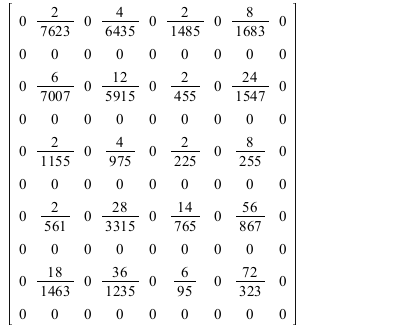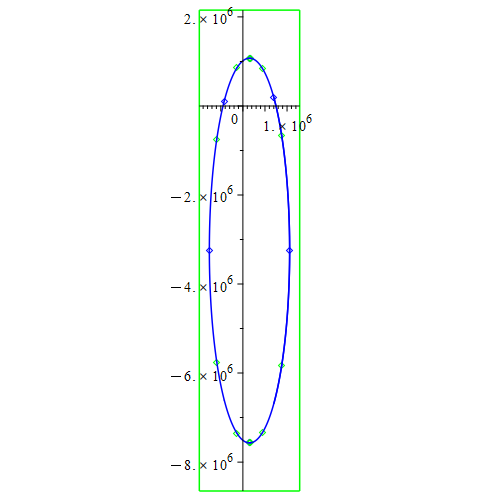Carl Love

## 25831 Reputation

10 years, 359 days
Himself
Wayland, Massachusetts, United States
My name was formerly Carl Devore.

## F3...

The hot-key for that (at least in Windows) is F3.

## SetOf...

Infinite sets (whether discrete or not) can be represented in Maple by using the inert SetOf constructor with a type-based membership criterion. Examples:

1. Since prime is a predefined type, the set of prime numbers can be represented as SetOf(prime).
2. There's no limitation to predefined types: odd is also a predefined type, so the set of odd primes can be represented via a constructed type as SetOf(And(odd, prime)).
3. #2 is mathematically the same as SetOf(odd) intersect SetOf(prime). Since Maple's type system is far more sophisticated than its property system (which SetOf is a part of), I prefer #2 because it puts most of the computation within the type system.

Your example starting sets are (where N is "natural numbers")

• A: natural numbers at least 20;
• B: {3*k - 1  |  k in N};
• C: {2*k + 1  |  k in N}.

These can be defined by (there are many alternative ways to construct the required types; I'm simply showing my favorites):

A:= SetOf(And(posint, Not(integer[1..20])));
B:= SetOf(And(posint, (-1) &under rcurry(mods, 3)));
C:= SetOf(And(odd, Not(1)));

The derived sets that you want are

• A intersect B intersect C;
• B minus C;
• A intersect (B minus C),

which can be constructed via

ABC:= A intersect B intersect C:  #or `intersect`(A, B, C)
`B\\C`:= B minus C:
`A(B\\C)`:= A intersect `B-C`:

They're still infinite sets of course, but they can be used for membership tests via the `in` infix operator, for example,

is(23 in ABC);
true

is(23 in `B\\C`);
false

Explicitly listed finite intersections can be made with select. For example the elements of the above 3 sets at most 100 can be listed via

(op@print~@map)(select, `in`, {\$1..100}, [ABC, `B\\C`, `A(B\\C)`]):
{23, 29, 35, 41, 47, 53, 59, 65, 71, 77, 83, 89, 95}
{2, 8, 14, 20, 26, 32, 38, 44, 50, 56, 62, 68, 74, 80, 86, 92, 98}
{26, 32, 38, 44, 50, 56, 62, 68, 74, 80, 86, 92, 98}

## Reassign, but don't redeclare, the paren...

A child class may reassign its parent's members, but it can't redeclare them (as localexport, static, etc.).  So, here is that principle applied to your example:

 > restart;
 > module ode_class() option object; local ode::`=`, x::symbol, y::symbol; export     #constructor     ModuleCopy::static:= proc(_self::ode_class, proto::ode_class, ode, func, \$)         (_self:-ode, _self:-y, _self:-x):=             if nargs=2 then (proto:-ode, proto:-y, proto:-x)             else (ode, op(0..1, func))             fi       end proc,    get_ode::static:= proc(_self, \$) ode end proc,    get_x::static:= proc(_self, \$) x end proc,    get_y::static:= proc(_self, \$) y end proc ; end module : module first_order_ode_class() option object(ode_class); local is_linear_ode::truefalse; export is_linear::static:= proc(_self, \$) is_linear_ode end proc;     #constructor     ModuleCopy:= proc(         _self::first_order_ode_class, proto::first_order_ode_class, ode, func, \$     )         (_self:-ode, _self:-y, _self:-x, _self:-is_linear_ode):=             if nargs=2 then (proto:-ode, proto:-y, proto:-x, proto:-is_linear_ode)             else (ode, op(0..1, func), false)             fi    end proc            end module :
 > ode:=Object(first_order_ode_class, diff(y(x),x)=sin(x), y(x));> ode:-get_ode(); ode:-is_linear()> type(ode, ode_class);> type(ode, first_order_ode_class);>

## See ?module,named...

The differences, which are fairly minor, are described on the help page ?module,named.

## Need to declare coordinates...

You need to declare your coordinate system when creating the vector field. Once that's done, DivergenceCurl, and other differential operators are simple commands (with no options needed):

with(VectorCalculus):
n:= VectorField(<cos(theta(x,y)), sin(theta(x,y)), 0>, cartesian[x,y,z]);
Divergence(n);
Curl(n);

The coordinate system in this case is cartesian[x,y,z].

## curry and rcurry...

You can probably do what you want by making collect's second argument a list of variable names such as [x,y] rather than a single variable such as x. I can't say for sure without seeing your example.

Nonetheless, it's good to learn a few fundamental commands that let you build what you want on the fly, and thus not need to remember all the idiosyncratic options (and their orders) of a vast number of not-so-fundamental commands. Two of those fundamental commands are curry and rcurry. They let you create a new function by pre-passing some (not all) arguments to an existing function (or command or procedure). The only difference between them is that curry pre-passes arguments starting with the 1st position, and rcurry pre-passes them ending with the last position (the r stands for "right"). In your case you could do:

a:= randpoly([x,y,z], dense);  #my example polynomial
collect(a, x, rcurry(collect, y));

You may include a "form" argument with either or both of those, and you may include a "func" argument with the inner collect by making it the last argument of the rcurry.

I use curry and rcurry so much that I have abbreviations for them defined in my initialization file:

`&<`:= curry;  `&>`:= rcurry;

Using those, the command becomes

collect(a, x, collect &> y);

## Can't solve for the constant...

Series solutions are theoretically infinite series for which (usually) only a finite number of terms can be determined. Let's say that the independent variable is x, the constant of integration is C, and the expansion point is a. Generally, C appears in every nonzero term. The only way to solve for C is to plug in x=a because this is the only thing that will make all terms of the infinite series (other than the 1st term). Plugging in some other value of x would only allow you to approximate using your finite number of generated terms.

## Matrix with initializer...

Although your notation for the 0 case isn't complete, this is (to my mind, at the moment) the only possible sensible interpretation of it: Do the nonzero cases as specified; all other cases are 0.

There are a vast number of ways to implement that interpretation in Maple. Here is one---an x n matrix:

m:= 10:  n:= 9:  #example values
M:= Matrix((m,n), (i,j)-> `if`(((m - i)*(n - j))::odd, i*j/(m^2 - i^2)/(n^2 - j^2), 0));For any integers m and i, both m + i and m - i have the same parity, so there's no need to specify "plus or minus". Also, i = m implies (m - i)::even, so i = m and j = n don't need to be listed as special cases.

## Nested elementwise operator...

It can be done like this:

(convert~)~(fasteners, unit_free)

This can be extended any number of levels, but the syntax forbids (I believe) ~~, so the parentheses around convert~ are required.

To see what's happening, observe

(f~)~([[a], [b]], c);

[[f(a, c)], [f(b, c)]]

## Naive division...

I doubt that any factorization algorithm could beat the average-case time of this naive division algorithm:

```p_log:= (n::And(integer, Not(0)), p::And(posint, Not(1)))->
local k:= 0, q:= n; do k++ until (q/= p)::fraction
:
#Example:
p_log(2^9*3^7*5^2, 3);
7
```

## Descartes' Rule of Signs...

It can be quickly done by hand (or with the assistance of Maple), and a tiny guess. Are you familiar with Descartes' Rule of Signs [Wikipedia link]? If a real univariate polynomial is sorted by degree (direction doesn't matter), you count its number of sign changes of its coefficients, ignoring coefficients that are 0. If that number is odd, it guarantees a positive root; if it's 0, it guarantees that there's not a positive root (and if it's another even number, then you can't tell). So, for your polynomial we check P(1, y) because x=1 is positive and it's easy to plug in by hand. The resulting polynomial in y has 1 sign change, which is odd, so there's a positive root (let's call it r) for y.  Thus (x= 1, y= r) is a positive root for P(x,y).

This can also be done by the Maple command

sturm(P(1,y), y, 0, infinity);

## Procedure-form input...

You have two choices for your input to Maximize (and other commands in package Optimization): The objective and constraints are either all expressions or all procedures; they can't be a mixture of expressions and procedures. Your f is a procedure, and your Cons is a set of expressions. The error message is telling you to make Cons into a set of procedures.

For this problem, you don't need to use procedure-form input. Tom's Answer shows how to do it with expression input. But if you do want to use procedure-form input (which is sometimes necessary), here is a way to do it:

 > restart :
 > Cons:= {x+x <= 1., x+x <= 2.} :
 > #The rest of this works independently of the number of variables or their names. # X:= [indets(Cons, name)[]];  #List all variables> NV:= nops(X);  #number of variables> Obj:= f(X);> #converts expressions to form required for procedure-form input: Proc:= rcurry(unapply, X): #
 > Optimization:-Maximize(     Proc(Obj),     (Proc@(lhs-rhs))~(Cons), #inequality constraints     (0..infinity) \$ NV,  #boundary constraints (just to show how it's done)     (* assume= nonnegative, *)  #redundant due to boundary constraints     initialpoint= [0.5 \$ NV] );>

Here's one of many possible interpretations of what you want. I like this one because it doesn't require foreknowledge of the number of meaningful values of S||i.

for i while (x:= S||(i+1))::And(algebraic, Not(name)) do S1+= x od;

## algcurves:-plot_real_curve...

A great command for plotting polynomial curves in two variables (even those of high degree) is algcurves:-plot_real_curve. It figures out all those little details, such as the ranges.

```algcurves:-plot_real_curve(
2.96736996560705e-12*p^2 + 1.31319840299485e-13*t^2 - 8.89549693662593e-7*p +
8.53128393394231e-7*t - 3.65558815509970e-30*p*t - 1,  # Don't use "= 0"
p, t,
scaling= constrained
);```Since your coefficients have 16 digits precision, it's worth checking if a higher Digits setting makes any difference. I did check that, but it made no difference perceivable to the naked eye.

If your attention is restricted to polynomials of degree 2 in 2 variables (aka "conic sections"), then there are formulas of the 6 coefficients to tell you almost anything you want to know about its geometry, such as whether it's an ellipse, and, if so, ranges of the variables.

## Change [] to ()...

Change all square brackets [] that are used algebraically within the equations to round.parentheses (). The [] that group the equation and IC together in the dsolve command should remain. Get rid of the simplify command for now.

﻿